International
Tables for
Crystallography
Volume A
Space-group symmetry
Edited by M. I. Aroyo

International Tables for Crystallography (2016). Vol. A, ch. 1.3, p. 22

## Section 1.3.1. Introduction

B. Souvigniera*

aRadboud University Nijmegen, Faculty of Science, Mathematics and Computing Science, Institute for Mathematics, Astrophysics and Particle Physics, Postbus 9010, 6500 GL Nijmegen, The Netherlands
Correspondence e-mail: souvi@math.ru.nl

### 1.3.1. Introduction

| top | pdf |

We recall from Chapter 1.2that an isometry is a mapping of the point spacewhich preserves distances and angles. From the mathematical viewpoint,is an affine space in which two points differ by a unique vector in the underlying vector space. The crucial difference between these two types of spaces is that in an affine space no point is distinguished, whereas in a vector space the zero vector plays a special role, namely as the identity element for the addition of vectors. After choosing an origin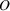, the points of the affine spaceare in one-to-one correspondence with the vectors ofby identifying a pointwith the difference vector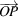.

A crystallographic space-group operation is an isometry that maps a crystal pattern onto itself. Since isometries are invertible and the composition of two isometries leaves a crystal pattern invariant as a whole if the two single isometries do so, the space-group operations form a group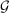, called a crystallographic space group.

As a mapping of points in an affine space, a space-group operation is an affine mapping and is thus composed of a linear mapping of the underlying vector space and a translation. Once a coordinate system has been chosen, space-group operations are conveniently represented as matrix–column pairs, whereis the linear part andthe translation part and a point with coordinatesis mapped to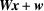(cf. Section 1.2.2).

A translation is a matrix–column pair of the form, whereis the unit matrix and all translations taken together form the translation subgroupof. The translation subgroup is an infinite group that forms an abelian normal subgroup of. The factor group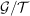is a finite group that can be identified with the group of linear parts ofvia the mapping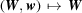, which simply forgets about the translation part. The group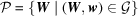of linear parts occurring inis called the point groupof.

The representation of space-group operations as matrix–column pairs is clearly adapted to the fact that space groups can be built from these two parts, the translation subgroup and the point group. This viewpoint will be discussed in detail in Section 1.3.3. It allows one to treat space groups in many aspects analogously to finite groups, although, due to the infinite translation subgroup, they are of course infinite groups.